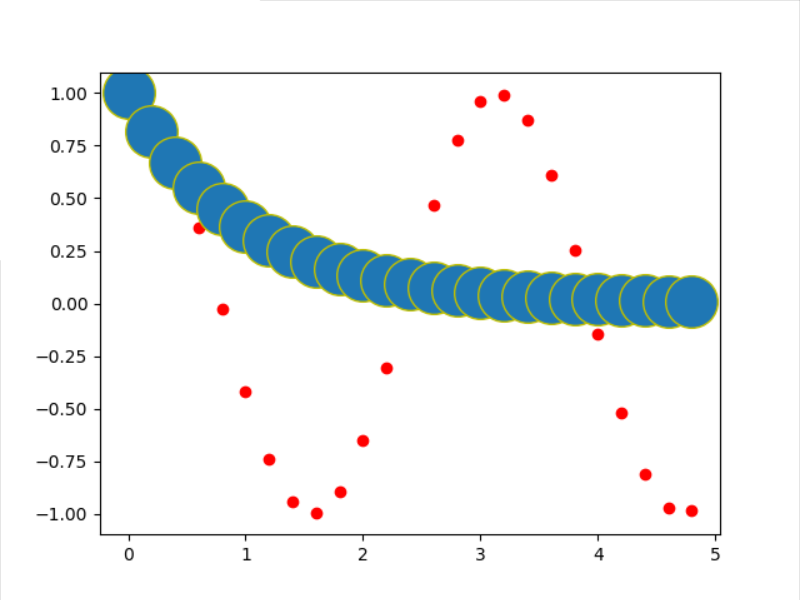# 创建函数的步骤

python是一种面向将对象的语言，所以与其说是函数更相对于是一种方法

``````def fname(t):
""" 这是一个自制的函数 """ #函数的帮助文档说明
s1 = np.cos(2 * t) #函数内部进行处理
e1 = np.exp(-t)
return s1, e1 #函数的返回值
``````
• 1
• 2
• 3
• 4
• 5

## 怎样调用该函数

``````import matplotlib.pyplot as plt
import numpy as np

def f(t):
""" 这是一个自制的函数 """
s1 = np.cos(2 * t)
e1 = np.exp(-t)
return s1, e1

help(f)
t1 = np.arange(0.0, 5.0, .2)
y1,y2= f(t1)
l = plt.plot(t1,y1,'ro')
l = plt.plot(t1,y2,'yo')
plt.setp(l, markersize=30)
plt.setp(l, markerfacecolor='C0')
plt.show()
``````
• 1
• 2
• 3
• 4
• 5
• 6
• 7
• 8
• 9
• 10
• 11
• 12
• 13
• 14
• 15
• 16
• 17
• 18
• 19f(t)

## 如何像MATLAB语言那样将函数单独存放在一个文件中

``````import numpy as np

def f(t):
""" 这是一个自制的函数 """
s1 = np.cos(2 * t)
e1 = np.exp(-t)
return s1, e1
``````
• 1
• 2
• 3
• 4
• 5
• 6
• 7
• 8

``````import mymodel as me
import numpy as np
import matplotlib.pyplot as plt

help(me.f)
t1 = np.arange(0.0, 5.0, .2)
y1,y2= me.f(t1)
l = plt.plot(t1,y1,'ro')
l = plt.plot(t1,y2,'yo')
plt.setp(l, markersize=30)
plt.setp(l, markerfacecolor='C0')
plt.show()
``````
• 1
• 2
• 3
• 4
• 5
• 6
• 7
• 8
• 9
• 10
• 11
• 12

## 总结：

python在当前文件中，建立函数的方法为 def

``````def f(t): #定义函数名
""" 这是函数解释文档 """
#添加一些处理语句
return s1 #返回一些变量
``````
• 1
• 2
• 3
• 4

python在其他文件中建立函数的方法：